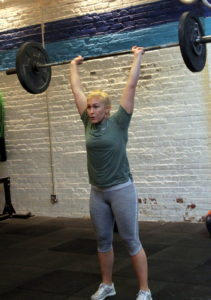## Wednesday, August 21, 2019

### Wednesday, August 21, 2019as many rounds as possible in 10 minutes:
7x swing @ 70/55-lbs.
7x box jump, 36″/30″ platform

### 1 Comment

1.–Scores–
Hari P.: 7 rounds + 7x swing
Charles B.: 6 rounds + 1x swing
Matt H.: 7 rounds + 5x box jump (55-lbs.)
Charlie K.: 8 rounds + 2x box jump (45-lbs.)
Borja G.: 10 rounds + 3x box jump (sub: pull-up)
Ben E.: 7 rounds + 3x swing (35-lbs.)
Zach D.: 9 rounds + 2x swing
Adrian A.: 7 rounds (45-lbs., 28″)
Al C.: 7 rounds + 2x swing
Jason Sc.: 7 rounds + 7x swing (55-lbs.)
Anu B.: 6 rounds + 1x box jump (55-lbs., 34″)
John Mc.: 7 rounds + 5x swing (35-lbs., 24″)
Nick W.: 7 rounds + 7x swing (55-lbs.)
Sean F.: 8 rounds + 1x box jump
Adrian P.: 4 rounds + 4x box jump (55-lbs., 28″)
Michael H.: 7 rounds (25-lb. dbs; sub: pull-up, 10 x weighted lunged)
Sarah D.: 9 rounds + 1x box jump (25-lbs., 20″)
Jin D.: 8 rounds + 1x box jump (45-lbs.)
Dave R.: 9 rounds + 3x swing
Kate G.: 10 rounds (35-lbs.)
Claire S.: 5 rounds (25-lbs., 20″)
Rebekka E.: 5 rounds
Seth B.: 7 rounds (55-lbs., 31″)
Lisa C.: 8 rounds + 5x box jump (45-lbs., 24″)
Buffy M.: 8 rounds + 5x box jump (35-lbs., 20″)
Alex M.: 11 rounds + 1x box jump (55-lbs., 30″)
Mel F.: 9 rounds + 4x swing
Christopher S.: 9 rounds + 3x box jump (35-lbs., 28″)
Matt M.: 8 rounds + 7x swing
Bill M.: 8 rounds + 2x box jump (45-lbs., 24″)
Allie R.: 8 rounds (45-lbs.)
Tommy W.: 7 rounds + 7x swing (45-lbs.)
John S.: 7 rounds + 2x swing (55-lbs.)
Christopher P.: 7 rounds (55-lbs.)
Daniel D.: 10 rounds + 5x swing (55-lbs., 30″; sub: russian swing)
Tarek K.: 6 rounds + 6x swing (45-lbs.)
Alli P.: 8 rounds + 4x box jump (45-lbs.)
Ralph B.: 9 rounds + 1x swing (55-lbs., 10-lbs.; sub: 10x med ball throw sit up)
Chris H.: 8 rounds + 5x box jump
Andy G.: 9 rounds + 7x swing (55-lbs.; sub: 10x sit-up)
Joya TD.: 8 rounds + 4x swing (45-lbs.)
Michail C.:7 rounds + 2x swing (45-lbs., 32″)
Rabeh G.: 9 rounds + 5x swing (20-lbs., 24″)
Katie G.: 7 rounds + 2x swing (35-lbs., 24″)
Peter Mc.: 9 rounds + 5x swing
Brian F.: 7 rounds + 2x box jump (35-lbs., 30″)
Kate V.: 8 rounds + 2x box jump (20-lbs., 20″)
Sergio L.: 7 rounds + 7x swing (35-lbs., 20″)
Dan C.: 7 rounds (55-lbs., 32″)
Mark Co.: 8 rounds (55-lbs., 30″)
David B.: 10 rounds + 5x body row (sub: body row, push up)
Aron S.: 7 rounds + 3x box jump (35-lbs., 30″)
Lily S.: 8 rounds + 6x med ball throw sit up (35-lbs.; sub: med ball throw sit up)
Amy S.: 8 rounds + 2x box jump
James W.: 7 rounds + 1x swing (45-lbs., 31″)
Tom G.: 10 rounds (20-lbs., 24″; sub: russian swing, step up)